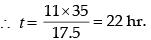Courses

# Test: Time And Distance - 2 (2015-2010)

## 15 Questions MCQ Test UPSC Topic Wise Previous Year Questions | Test: Time And Distance - 2 (2015-2010)

Description
This mock test of Test: Time And Distance - 2 (2015-2010) for UPSC helps you for every UPSC entrance exam. This contains 15 Multiple Choice Questions for UPSC Test: Time And Distance - 2 (2015-2010) (mcq) to study with solutions a complete question bank. The solved questions answers in this Test: Time And Distance - 2 (2015-2010) quiz give you a good mix of easy questions and tough questions. UPSC students definitely take this Test: Time And Distance - 2 (2015-2010) exercise for a better result in the exam. You can find other Test: Time And Distance - 2 (2015-2010) extra questions, long questions & short questions for UPSC on EduRev as well by searching above.
QUESTION: 1

### Two pipes A and B can independently fill a tank completely in 20 and 30 minutes respectively. If both the pipes are opened simultaneously, how much time will they take to fill the tank completely? 

Solution:

Time taken by both tanks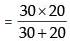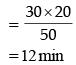QUESTION: 2

### Two cities A and B are 360 km apart. A car goes from A to B with a speed of 40 km/hr and returns to A with a speed of 60 km/hr. What is the average speed of the car? 

Solution:

Average speed of two cars at a speed V1 and V2 km/hr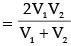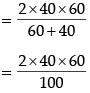= 48 km/hr

QUESTION: 3

### In a 500 metres race, B starts 45 metres ahead of A, but A wins the race while B is still 35 metres behind. What is the ratio of the speeds of A to B assuming that both start at the same time? 

Solution:

Total distance = 500m
Distance covered by A = 500m
Distance covered by B = 500 – 45 – 35
= 500 – 80
= 420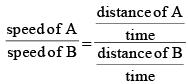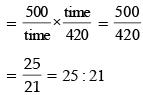QUESTION: 4

A worker reaches his factory 3 minutes late if his speed from his house to the factory is 5 km/hr, if he walks at a speed of 6 km/hr then he reaches the factory 7 minutes early. The distance of the factory from his house is



Solution:

Let the distance is x km
ATQ,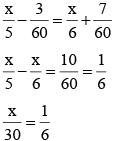∴ x = 5 km

QUESTION: 5

A and B decide to travel from place X to place Y by bus. A has ₹ 10 with him and he finds that it is 80% of the bus fare for two persons. B finds that he has ₹ 3 with him and hands it over to A. In this context, which one of the following statements is correct?



Solution:

Let cost of 1 ticket is ₹ x.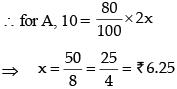Now B gives ₹ 3 to A.
So, A has 13 ₹.
Cost of 2 tickets = ₹ 12.50, which is more than enough to buy 2 tickets.
∴  (a) Rules out
(b) is clearly ruled out
(c) is true, because after buying 2 tickets.
A is left with ₹ 13 – 12.50 = 50 paise

QUESTION: 6

A straight line segment is 36 cm long. Points are to be marked on the line from both the end points. From each end, the first point is at a distance of 1cm from the end, the second point is at a distance of 2 cm from the first point and the third point is at a distance of 3 cm from the second point and so on. If the points on the ends are not counted and the common points are counted as one, what is the number of points?



Solution: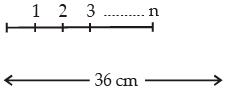∴ 1 + 2 + 3 + ........ n = 36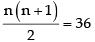n(n + 1) = 72
⇒ n = 8
∴ Leaving the end points the no. of points starting from A is 7.
Similarly starting from B, the no. of points will be 7. And also no. of the points between A & B will coincide.
∴ Total no. of points
= 7 + 7 = 14

QUESTION: 7

Two cars start towards each other, from two places A and B which are at a distance of 160 km. They start at the same time 08 :10 AM. If the speeds of the cars are 50 km and 30 km per hour respectively, they will meet each other at



Solution: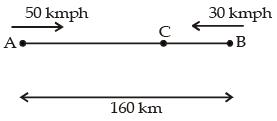Suppose the cars meet at point C after ‘t’ hrs.
∴ AC = 50 t and BC = 30 t
∴ 50 t + 30 t = 160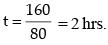So, the cars will meet at 10 : 10 AM

QUESTION: 8

Location of B is north of A and location of C is east of A. The distances AB and AC are 5 km and 12 km respectively. The shortest distance (in km) between the locations B and C is



Solution: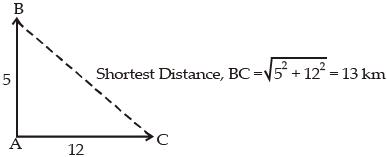QUESTION: 9

A train travels at a certain average speed for a distance of 63 km and then travels a distance of 72 km at an average speed of 6 km/hr more than its original speed. If it takes 3 hours to complete the total journey, what is the original speed of the train in km/hr?



Solution:

Let the original speed = x
Total time taken = 3 hr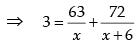⇒ (x) (x + 6) = 21(x + 6) + 24(x)
⇒ x2 – 39x – 126 = 0
⇒ x2 – 42x + 3x = 126 = 0
⇒ (x – 42) (x + 3) = 0
x = 42, x = – 3
x = – 3 is rejected.
Hence, original speed was 42 km/hr.

QUESTION: 10

A thief running at 8 km/hr is chased by a policeman whose speed is 10 km/hr. If the thief is 100 m ahead of the policeman, then the time required for the policeman to catch the thief will be



Solution:

If we consider the difference of speeds, policeman is 2 km/hr leading speed and he can catch the thief at 100 m ahead by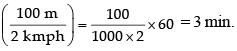QUESTION: 11

Four cars are hired at the rate of ₹ 6 per km plus the cost of diesel at ₹ 40 a litre. In this context, consider the details given in the following table: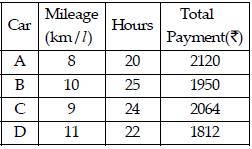Which car maintained the maximum average speed?



Solution:

Let the distances travelled by cars A, B, C and D be a, b, c and d respectively.
Then, the total payment for car A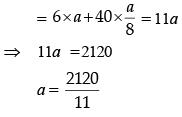Hence, average speed of ‘A’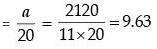For B, total payment for car B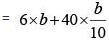⇒ 10b = 1950
∴ b = 195
Hence, average speed of ‘B’ = b/25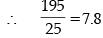For C, total payment for car C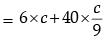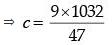Hence averge speed by ‘C’ = c/24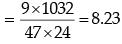For D, total payment for car D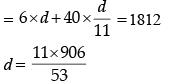Hence, the average speed of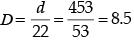All speeds except A are less than 9. Hence it’s greatest value.

QUESTION: 12

A person can walk a certain distance and drive back in six hours. He can also walk both ways in 10 hours. How much time will he take to drive both ways?



Solution:

2-way walk = 10 hrs
∴ 1-way walk = 5 hrs
1-way walk + 1 way drive = 6 hrs
∴ 1 way drive = 6 – 5 = 1 hr
Hence, two way drive take 2 hrs.

QUESTION: 13

Mr. Kumar drives to work at an average speed of 48 km per hour. The time taken to cover the first 60% of the distance is 10 minutes more than the time taken to cover the remaining distance. How far is his office?



Solution: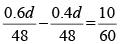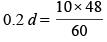∴ d = 40 km

QUESTION: 14

If a bus travels 160 km in 4 hours and a train travels 320 km in 5 hours at uniform speeds, then what is the ratio of the distances travelled by them in one hour?



Solution:

Distance covered by a bus in 4 hours = 160 km.
∴ Distance covered by a bus in 1 hour  = 160/4 = 40 km
Further, Distance covered by a train in 5 hours = 320 km
∴  Distance covered by a train in 1 hour = 320/5 = 64km
Required ratio  = 40/64 = 5/8 = 5:8

QUESTION: 15

Three men start together to travel the same way around a circular track of 11 km. Their speeds are 4, 5.5 and 8 kmph respectively. When will they meet at the starting point for the first time?



Solution:

Let the minimum time be t, when they meet at the starting point for the first time. So, the net distance covered must be a multiple of 11.
Now, (4 + 5.5 + 8) t = 11 (n)
where, n is an integer.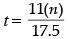minium value of n is 35, so that we can get an appropriate value of time.